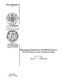## Performance Evaluation and Optimization of Parallel Systems with Synchronization

 dc.contributor.advisor Makowski, A. en_US dc.contributor.author Gun, Levent en_US dc.contributor.department ISR en_US dc.date.accessioned 2007-05-23T09:44:45Z dc.date.available 2007-05-23T09:44:45Z dc.date.issued 1989 en_US dc.description.abstract This thesis considers synchronization issues such as resequencing and fork/join in parallel architectures. The discussion is carried out in the context of K parallel single server queues with general servers where jobs are subject to resequencing. Both performance evaluation and optimal routing problems are addressed for such systems. In the first part, Poisson arrivals are assumed to be randomly allocated to different queues according to a Bernoulli switch. The distributions of the various delays in the system are obtained by sample path arguments. The problem of choosing the switching probabilities that minimize the average end-to-end delay is considered. In addition to obtaining exact results in some cases, simple but accurate approximations are provided when the service time distributions are exponential. The simple form of these approximations is then utilized to solve the optimization problem in the case when the service parameters are unknown, and a simple stochastic approximation algorithm is proposed. When the servers are all identical, several useful asymptotic results are obtained as K increases to infinity. Various stochastic monotonicity and convexity results are also provided for this parallel system. In the second part, the dynamic optimization of the same model is investigated under more general assumptions for the arrival process. The resequencing problem is combined with a fork/join problem, where the incoming packets are broken into smaller subpackets for processing at different queues. The problem of finding the optimal allocation policy that minimizes the average discounted and the long-run average costs is formulated as a Markov Decision problem, where the cost-per-stage is taken as the end-to-end delay of each packet. In both cases, the optimal policy is identified as the one that drives the workload in each queue to a balanced configuration as quickly as feasible. en_US dc.format.extent 3534501 bytes dc.format.mimetype application/pdf dc.identifier.uri http://hdl.handle.net/1903/4944 dc.language.iso en_US en_US dc.relation.ispartofseries ISR; PhD 1989-3 en_US dc.subject Communication en_US dc.subject Signal Processing Systems en_US dc.title Performance Evaluation and Optimization of Parallel Systems with Synchronization en_US dc.type Dissertation en_US
##### Original bundle
Now showing 1 - 1 of 1